# RS Aggarwal Solutions for Class 9 Chapter 1: Number Systems Exercise 1G

## RS Aggarwal Solutions for Class 9 Maths Exercise 1G PDF

RS Aggarwal Solutions for Class 9 is the best reference material which can be used by the students to boost their exam preparation. The solutions are accurate with explanations in simple language prepared by our faculties having much experience in Mathematics. By practising the exercises regularly the students can make use of the PDF to clear their doubts that would arise while solving. The students can do a better analysis while solving the problems so that mistakes are not repeated in the exams. RS Aggarwal Solutions for Class 9 Maths Chapter 1 Number Systems Exercise 1G are provided here.

## RS Aggarwal Solutions for Class 9 Chapter 1: Number Systems Exercise 1G Download PDF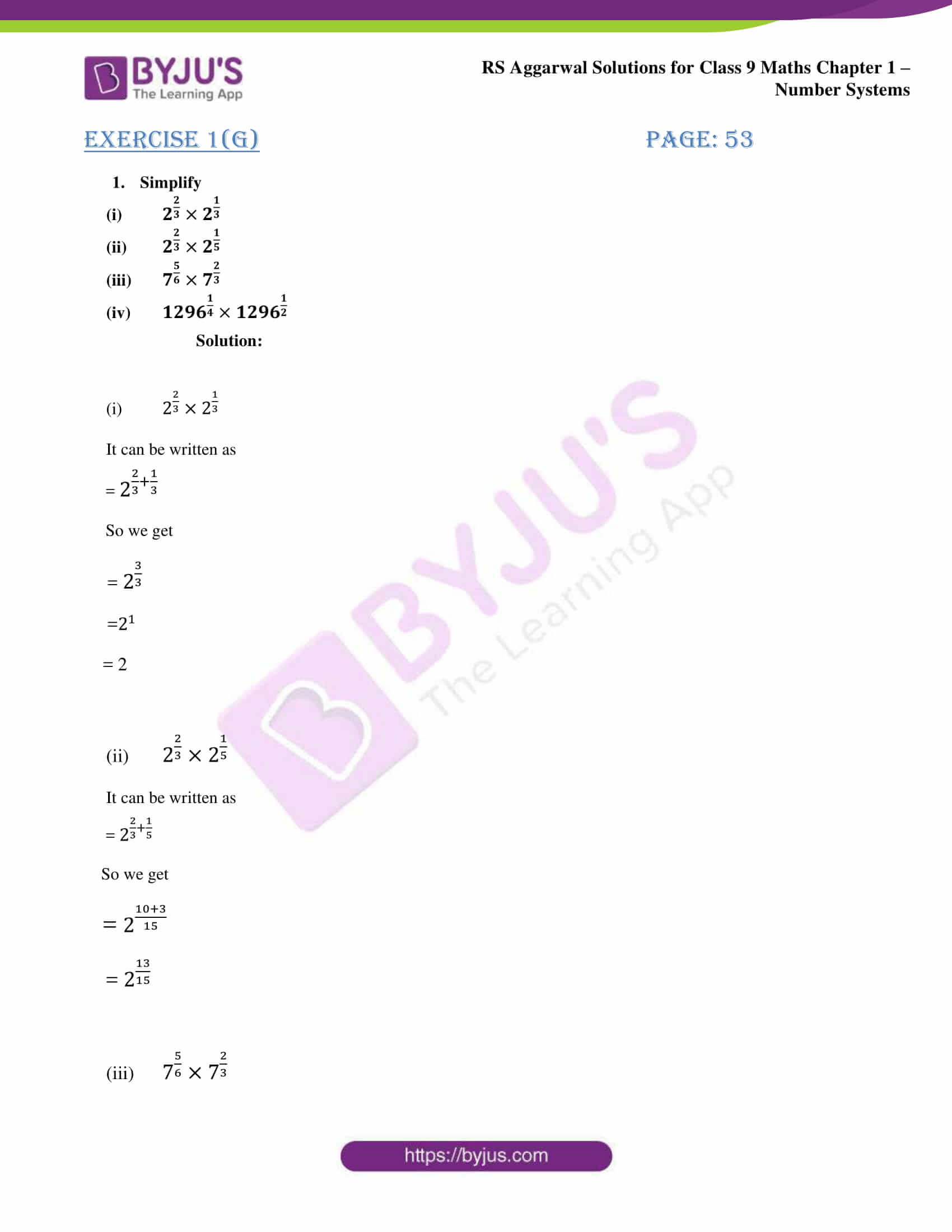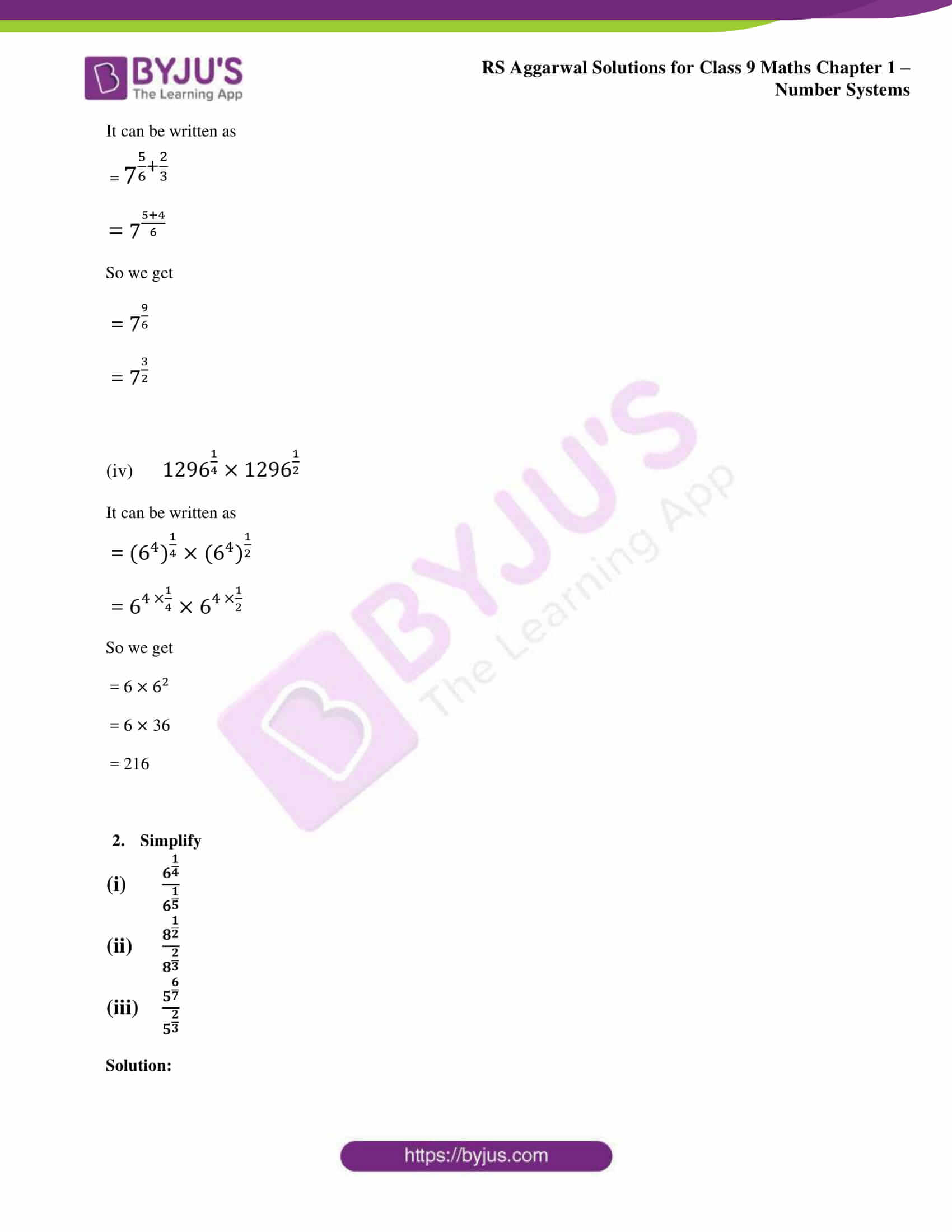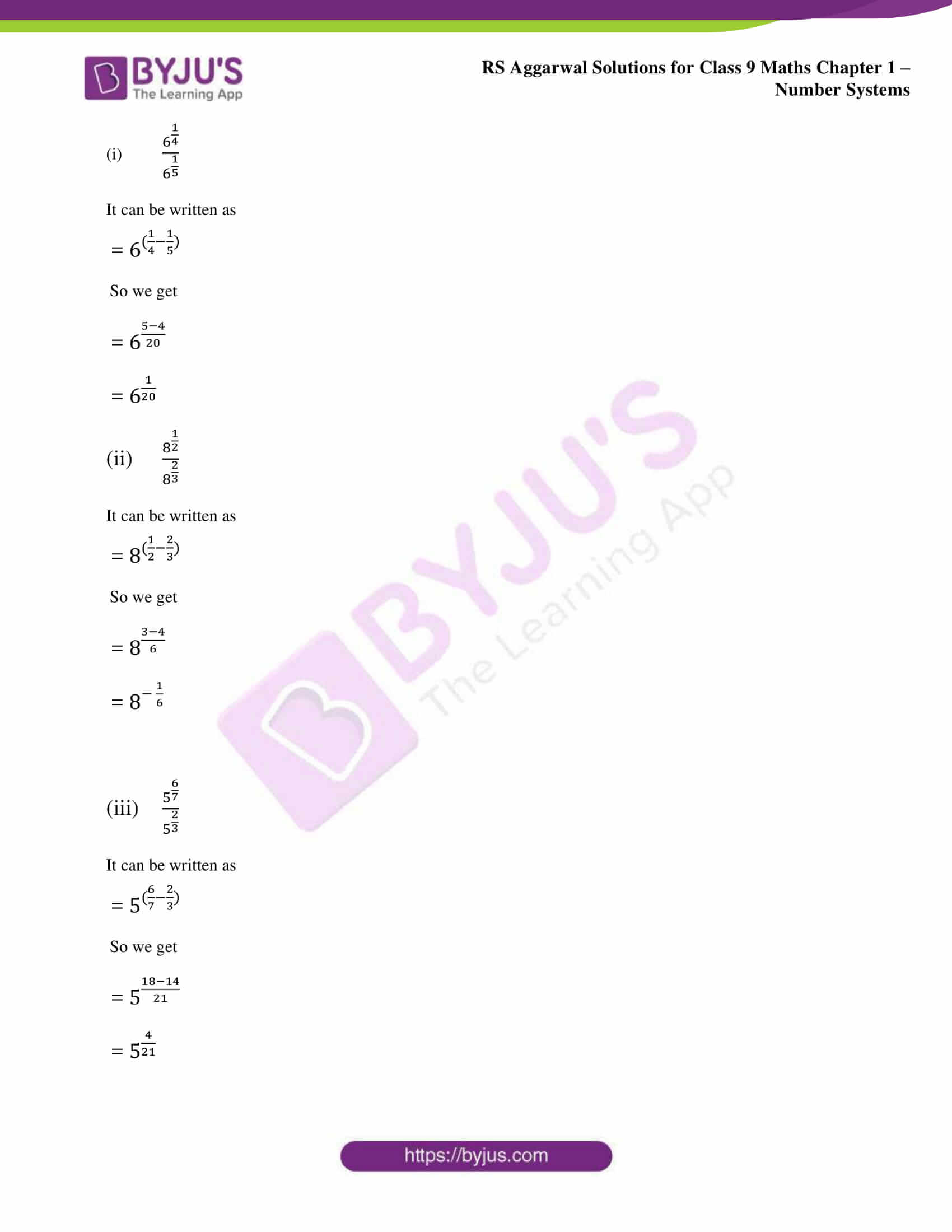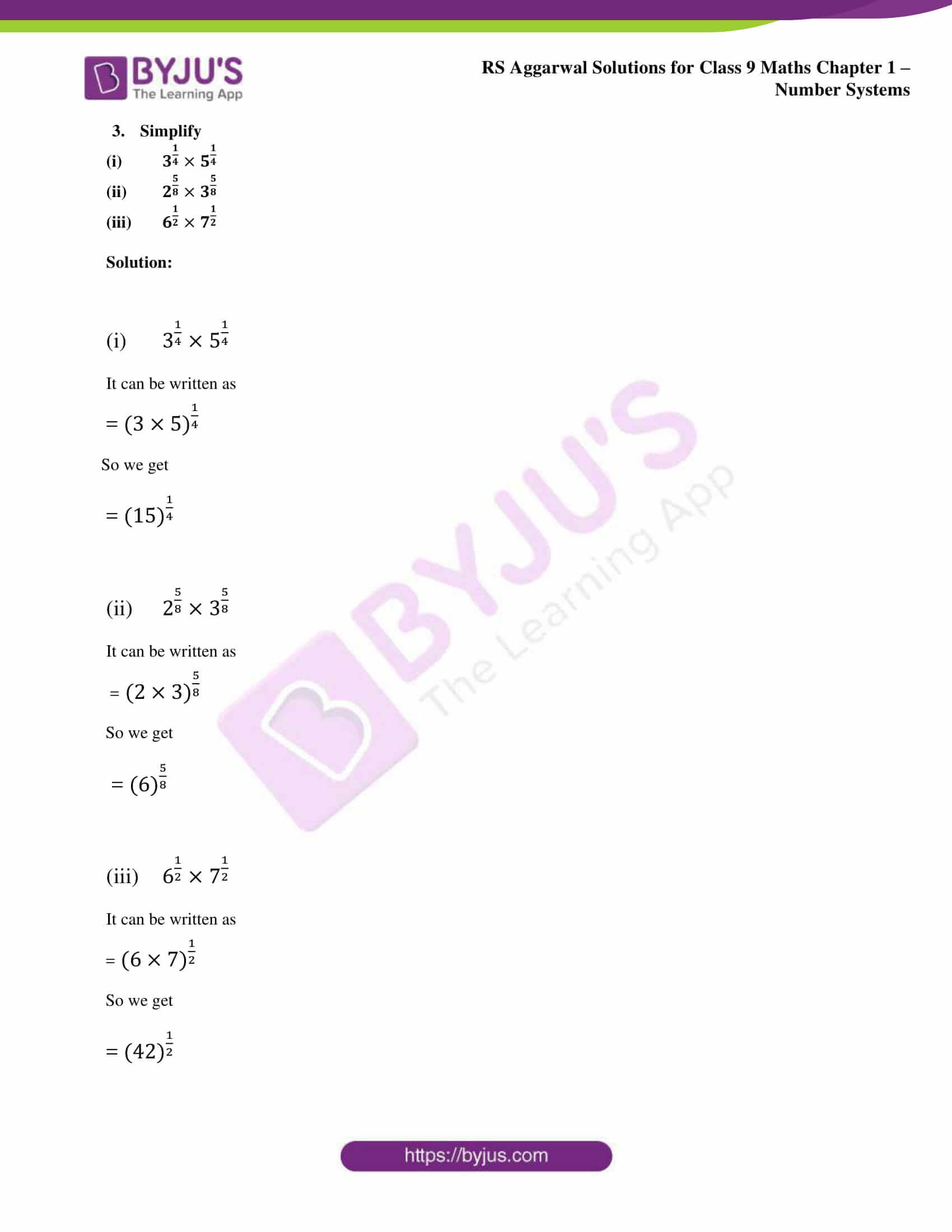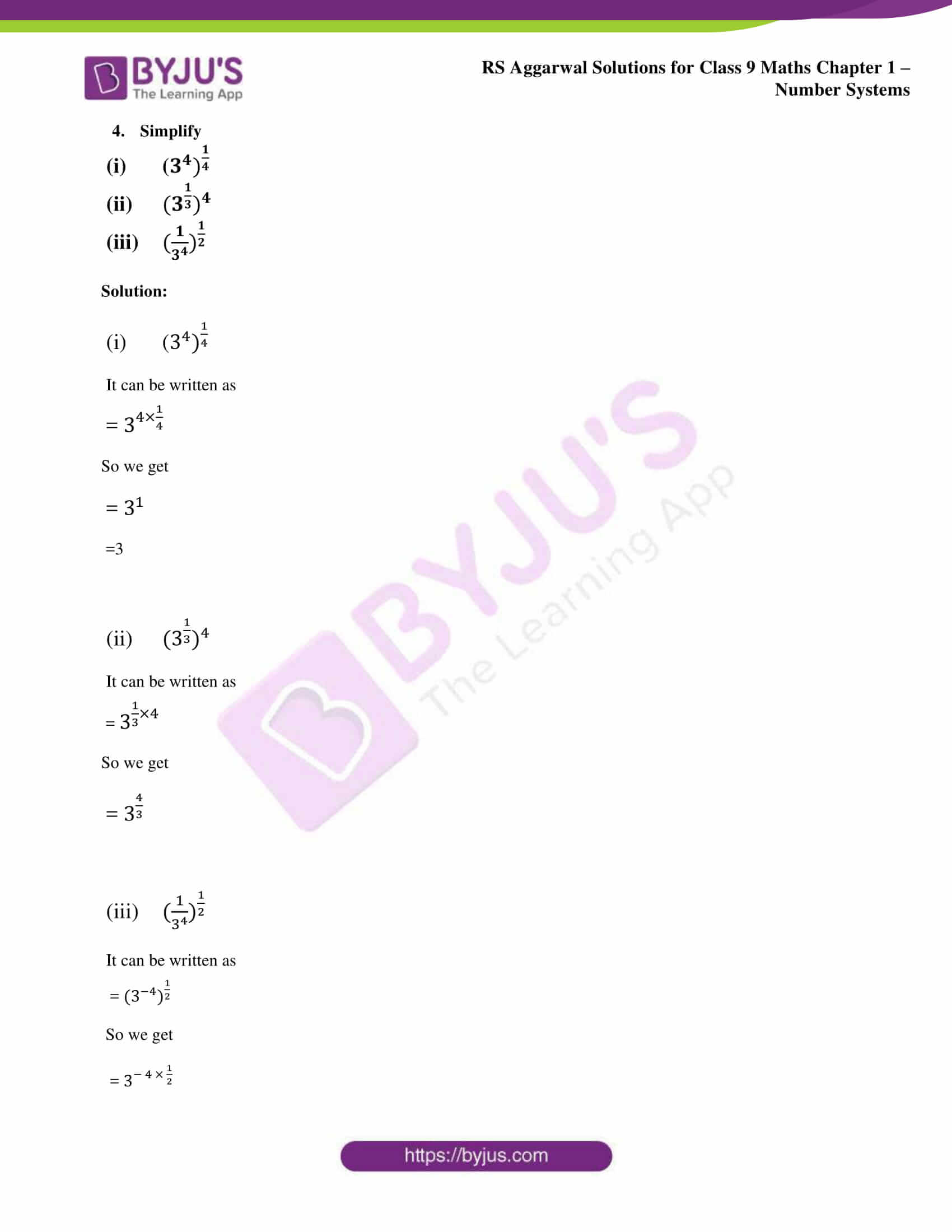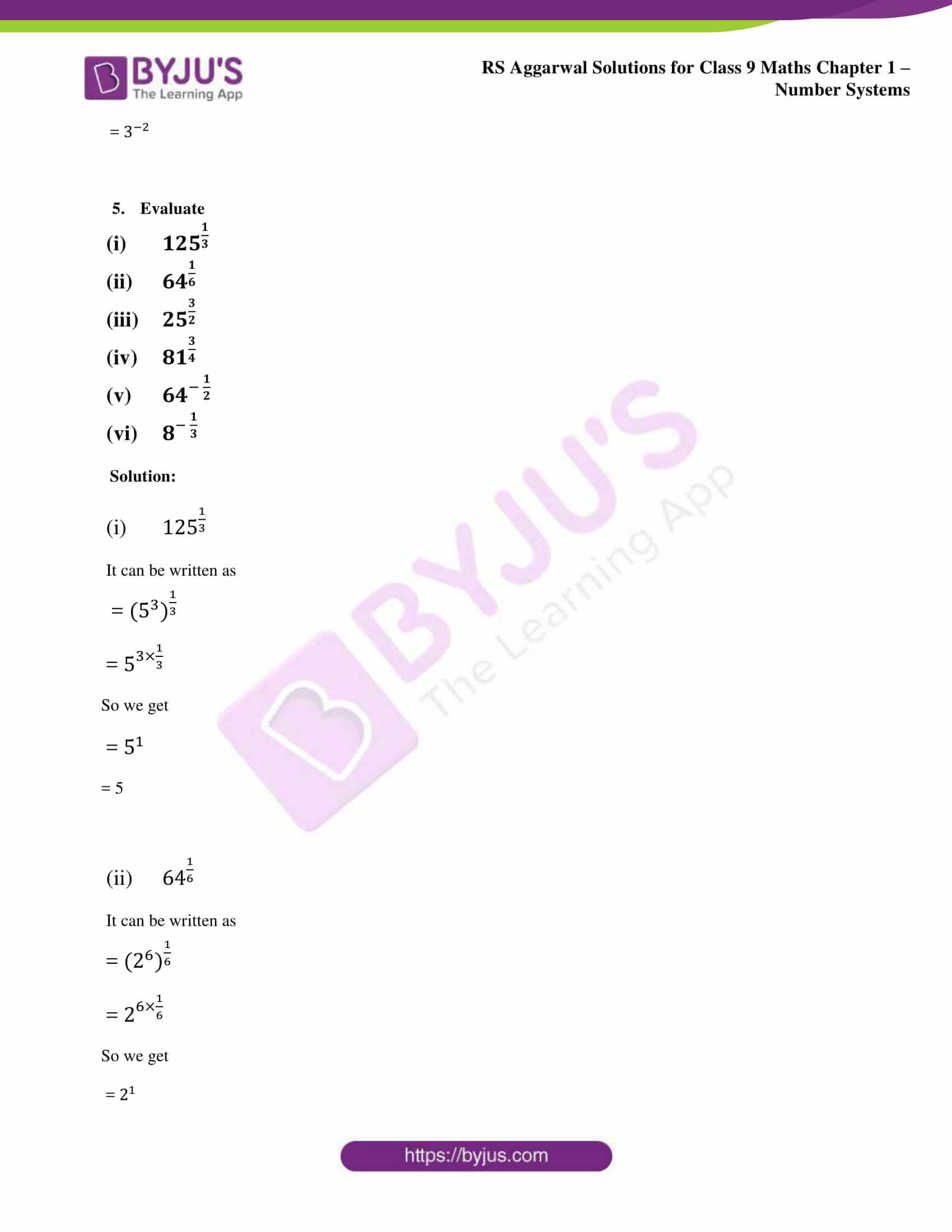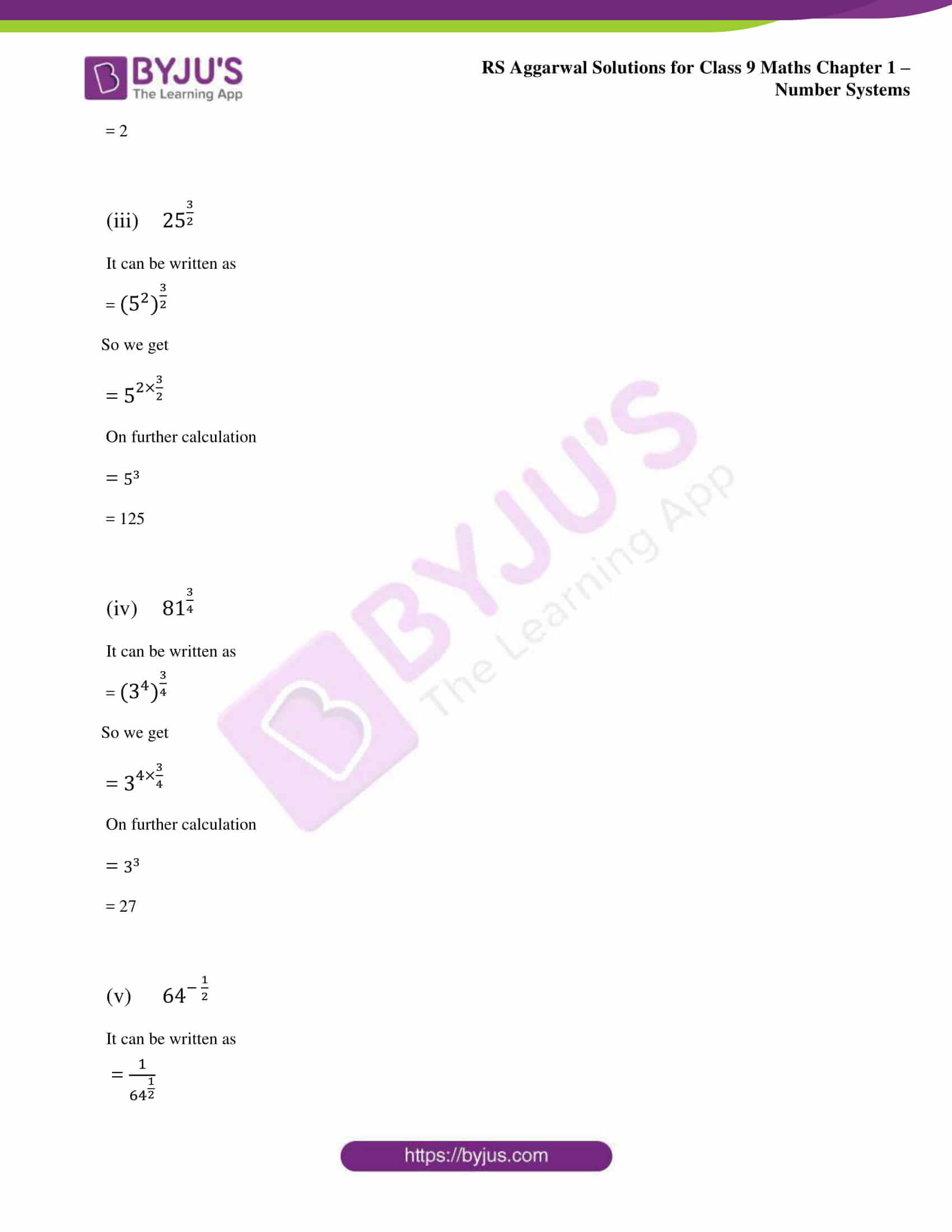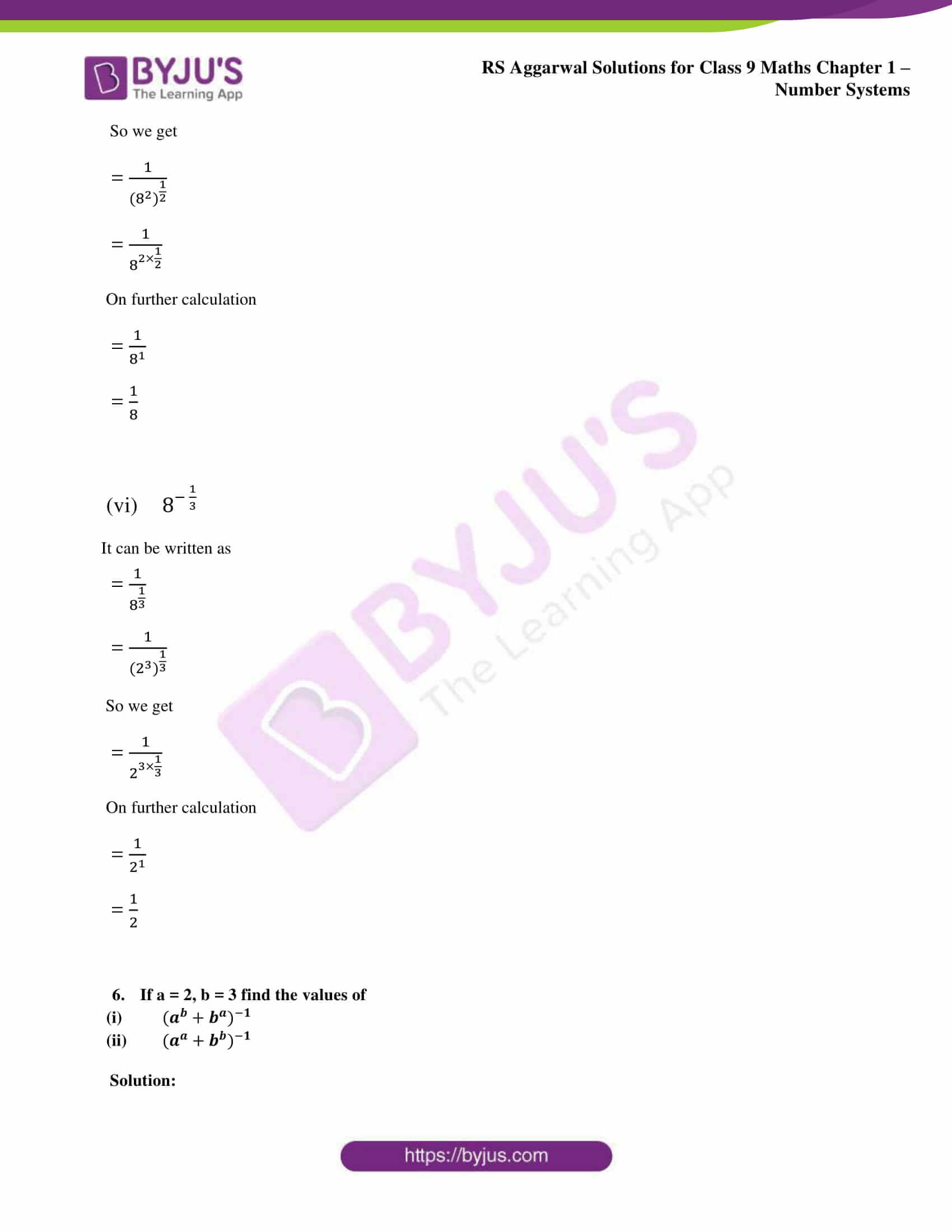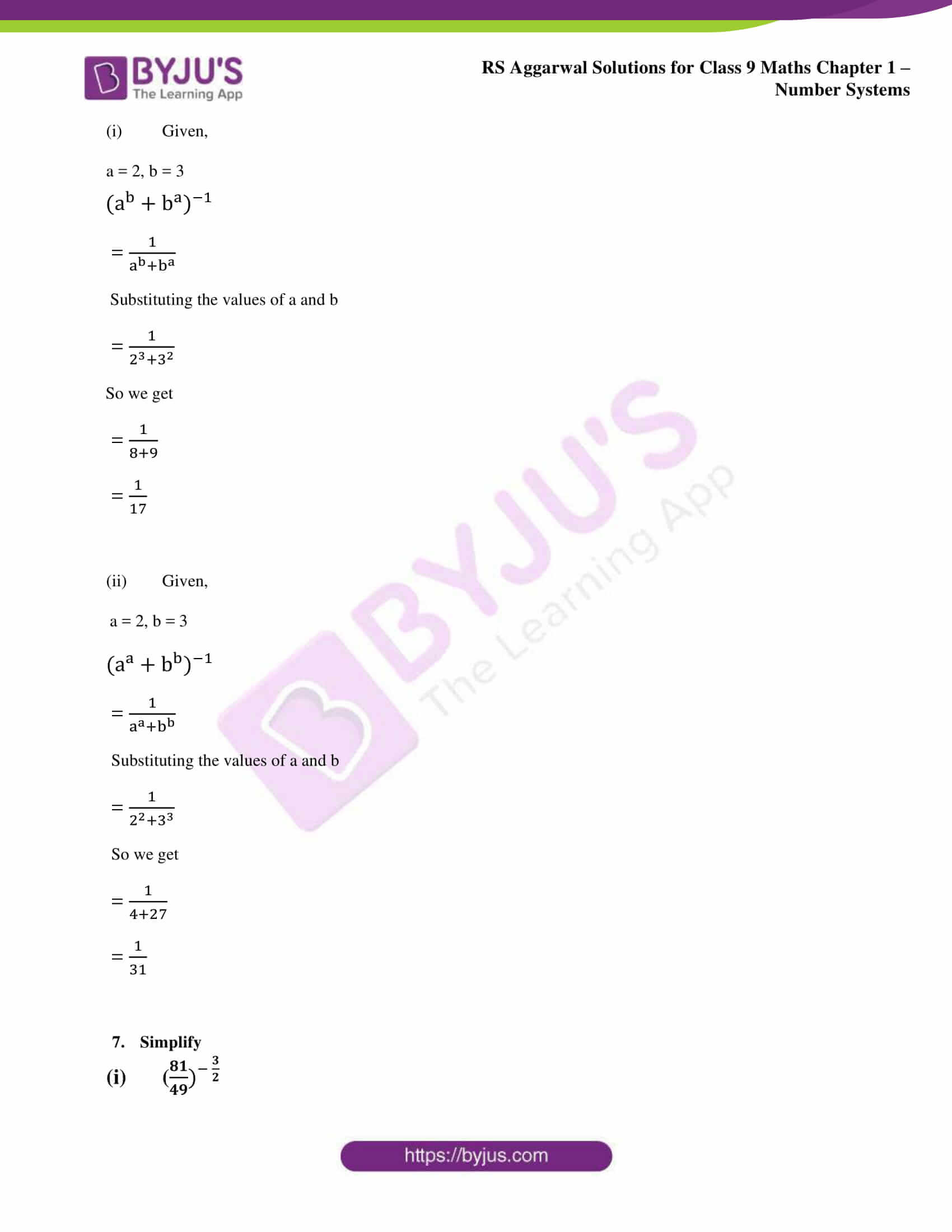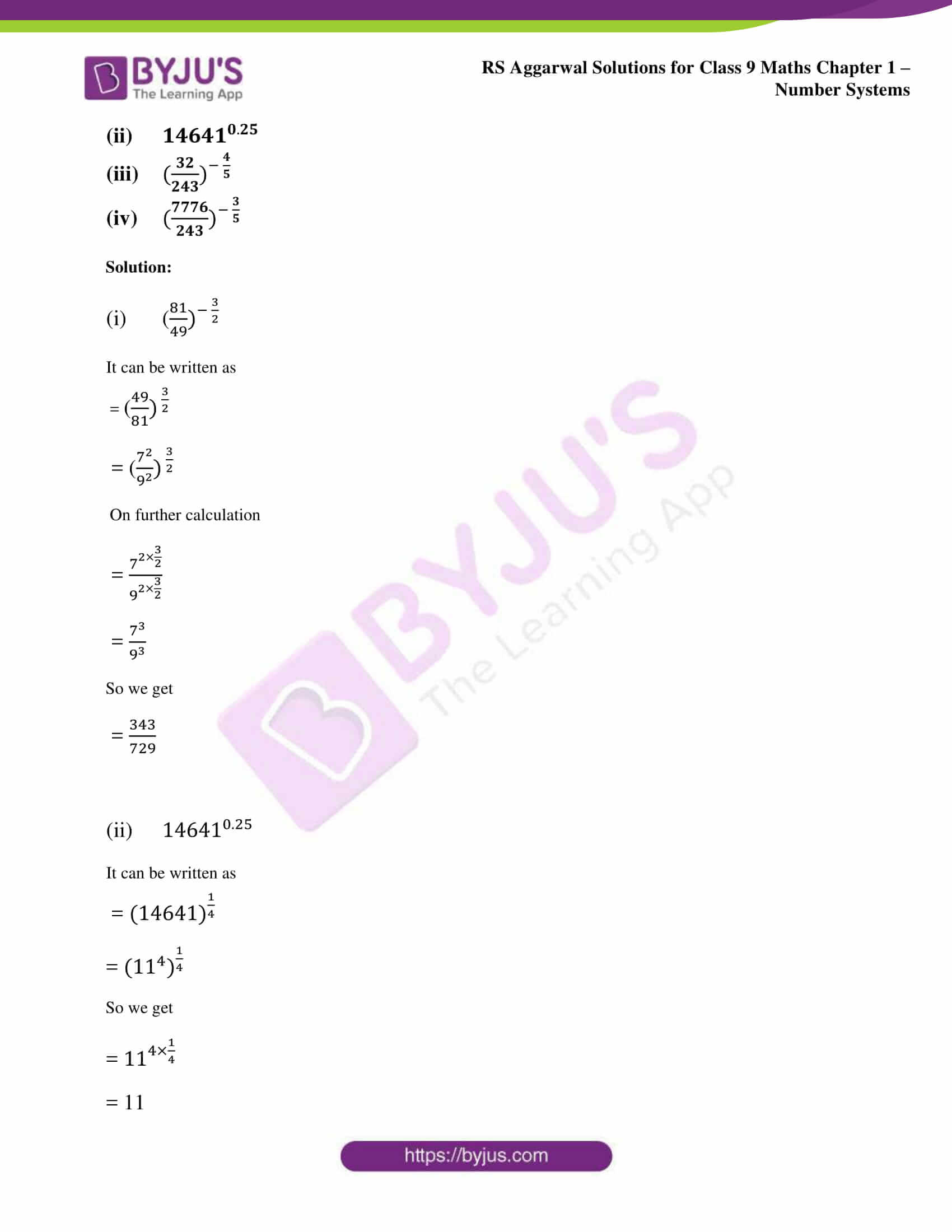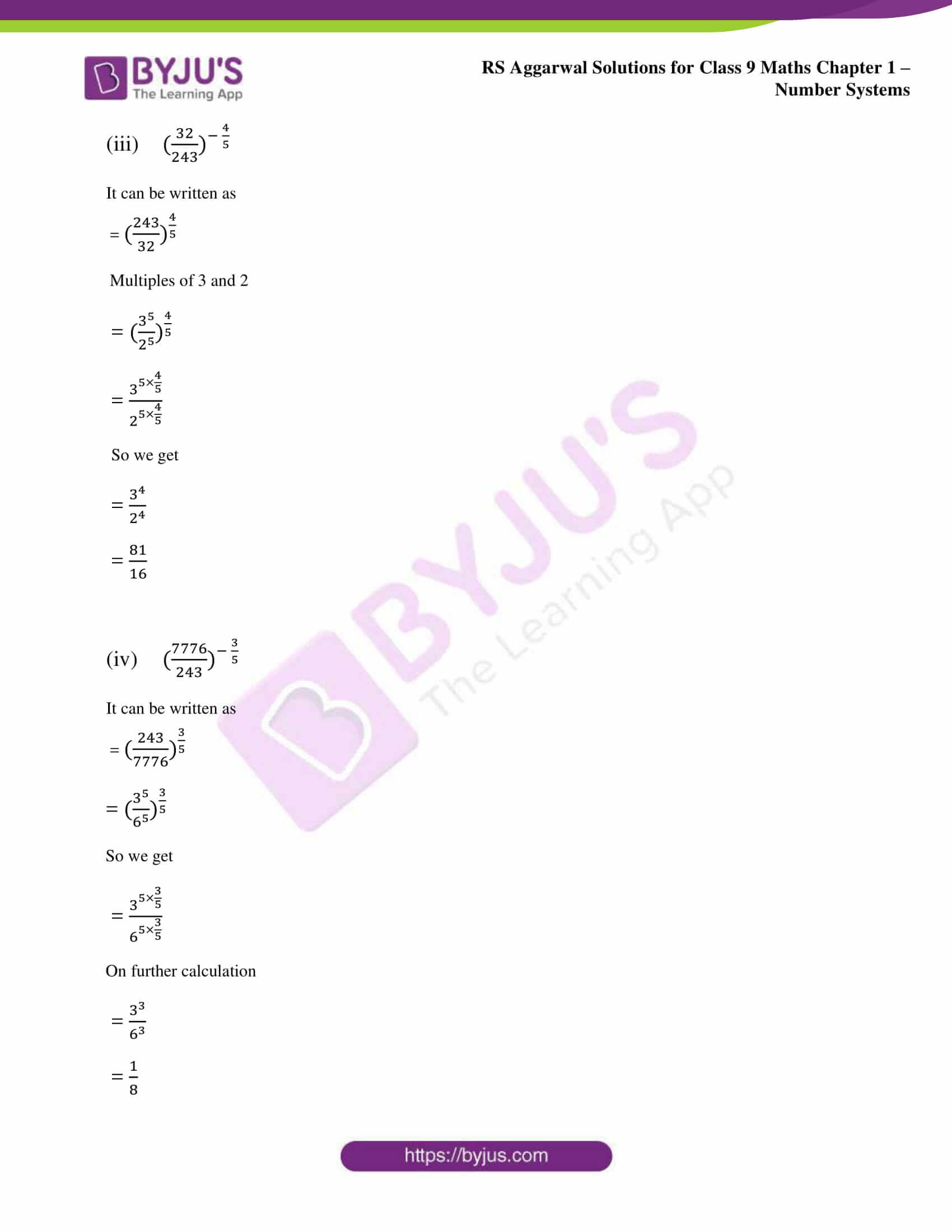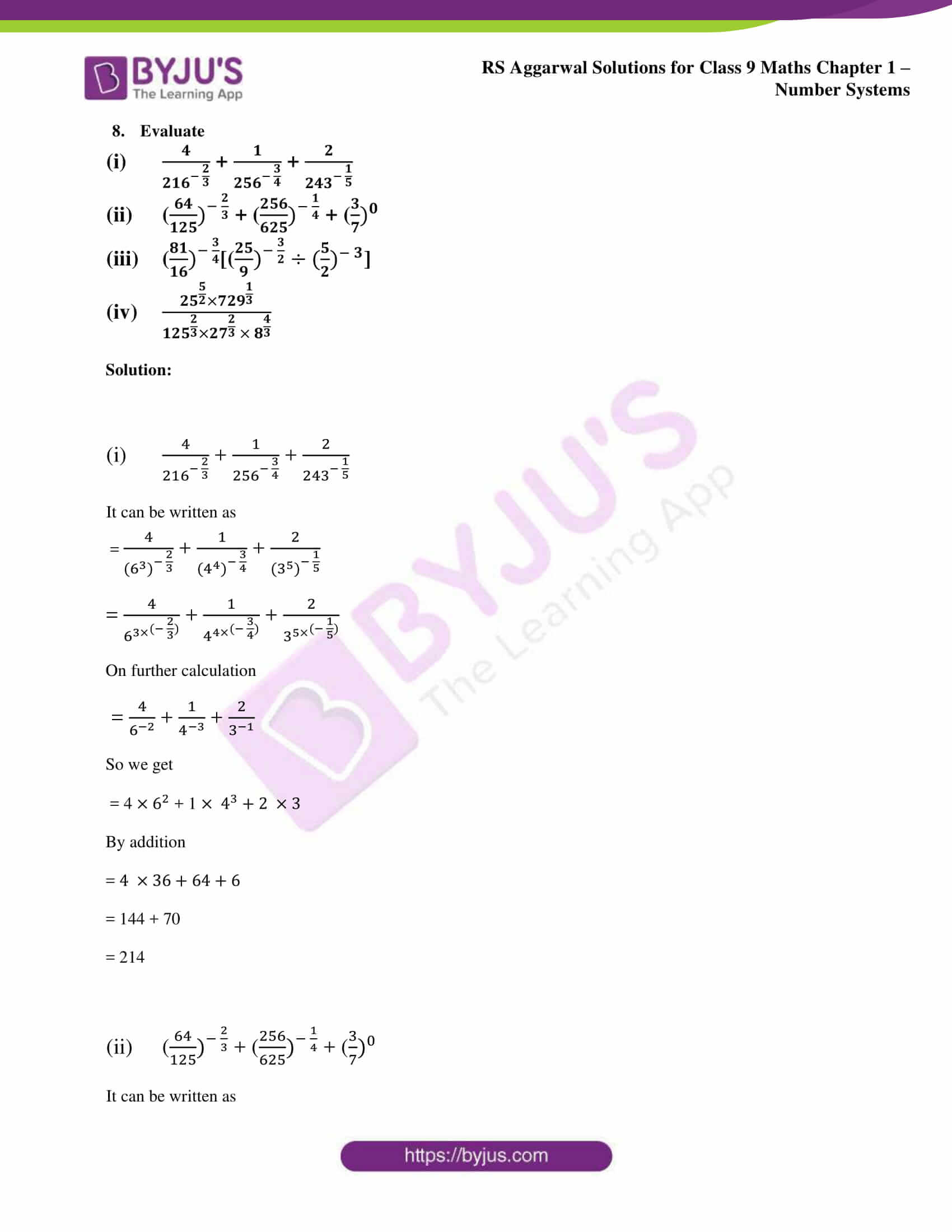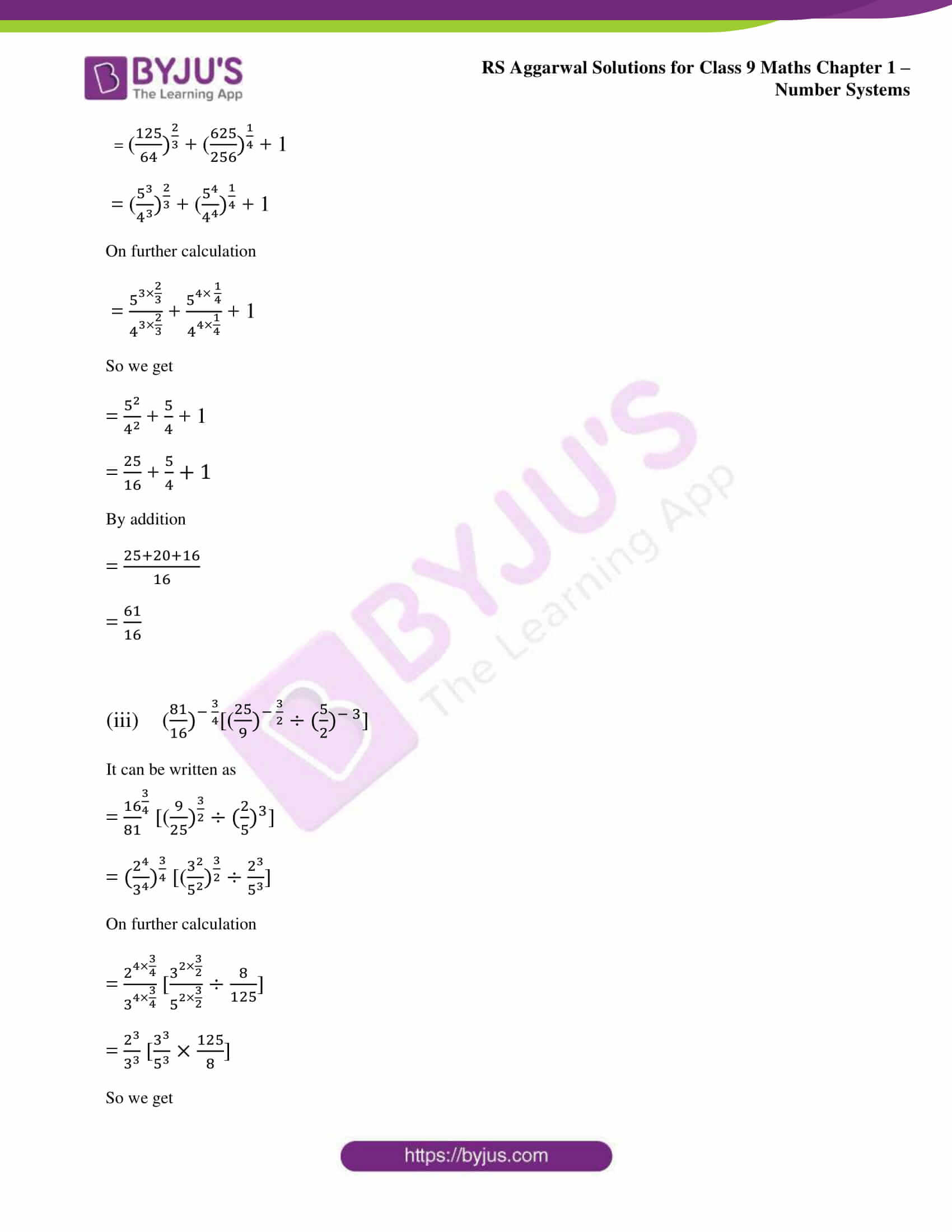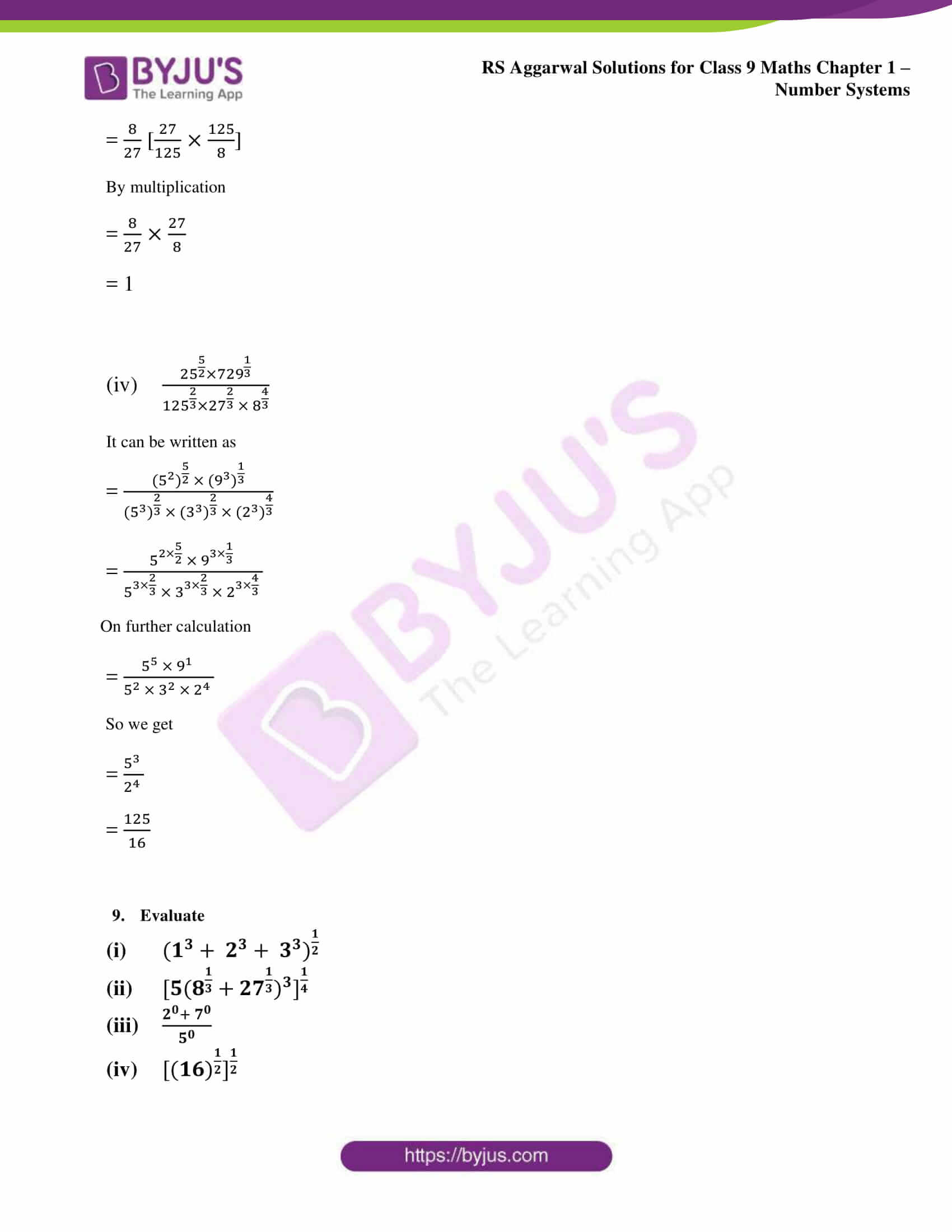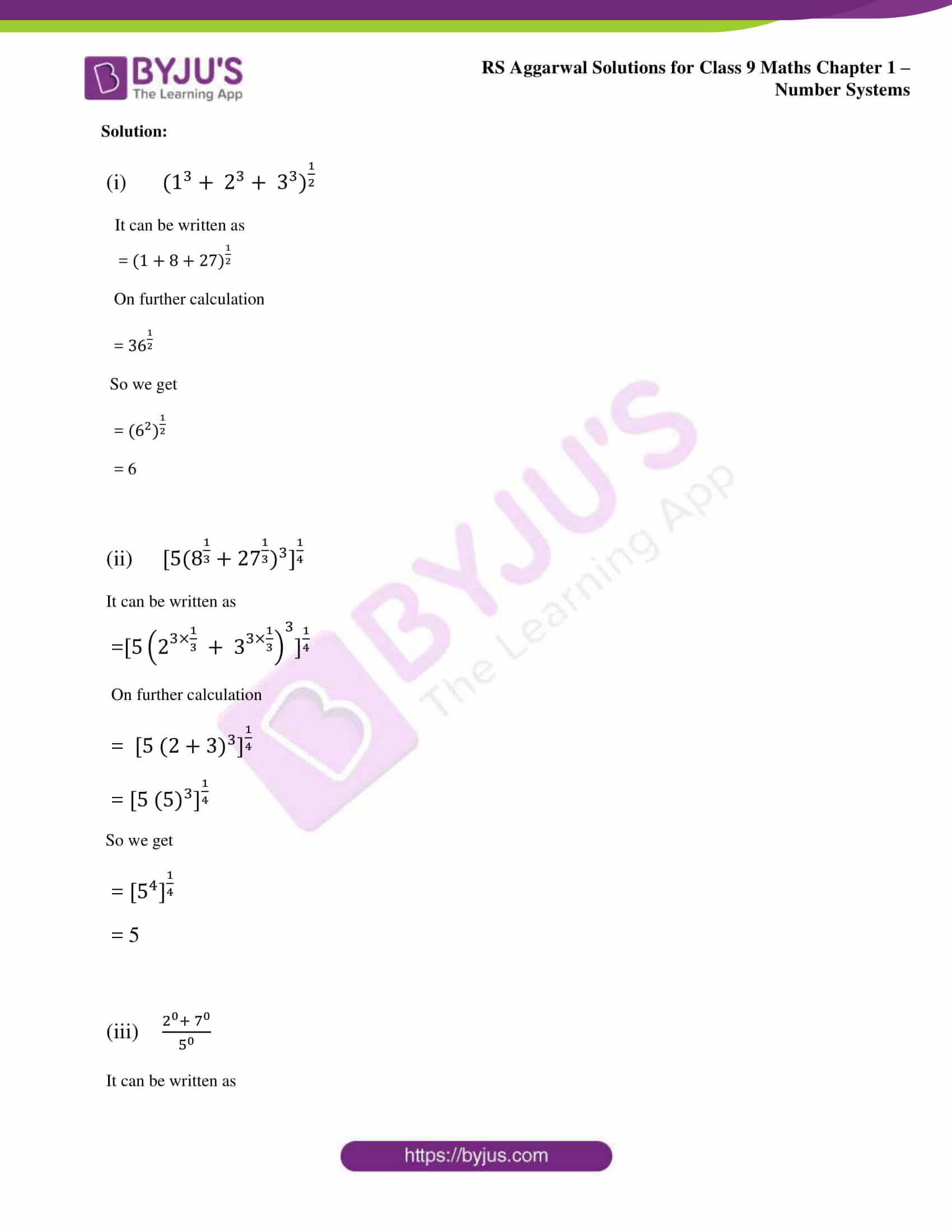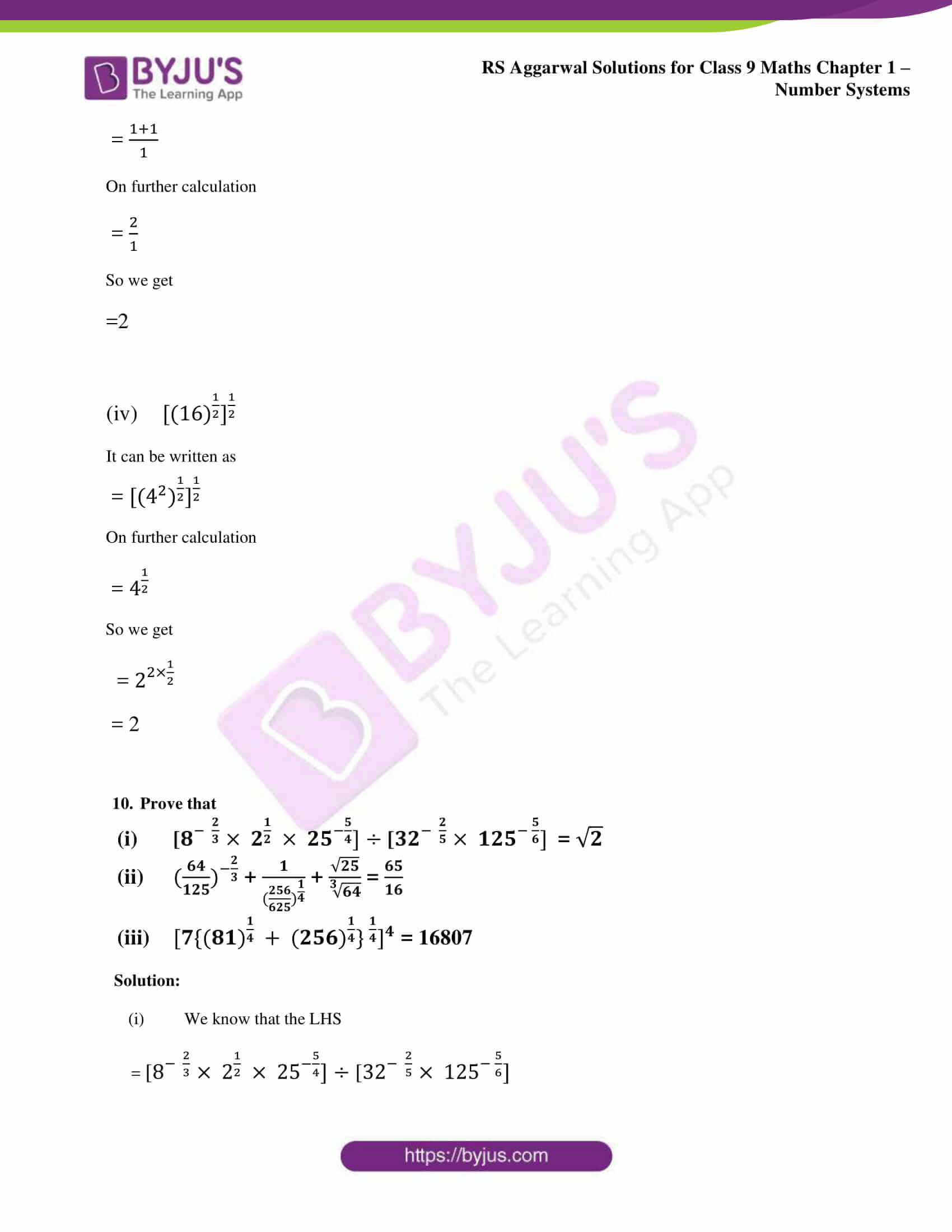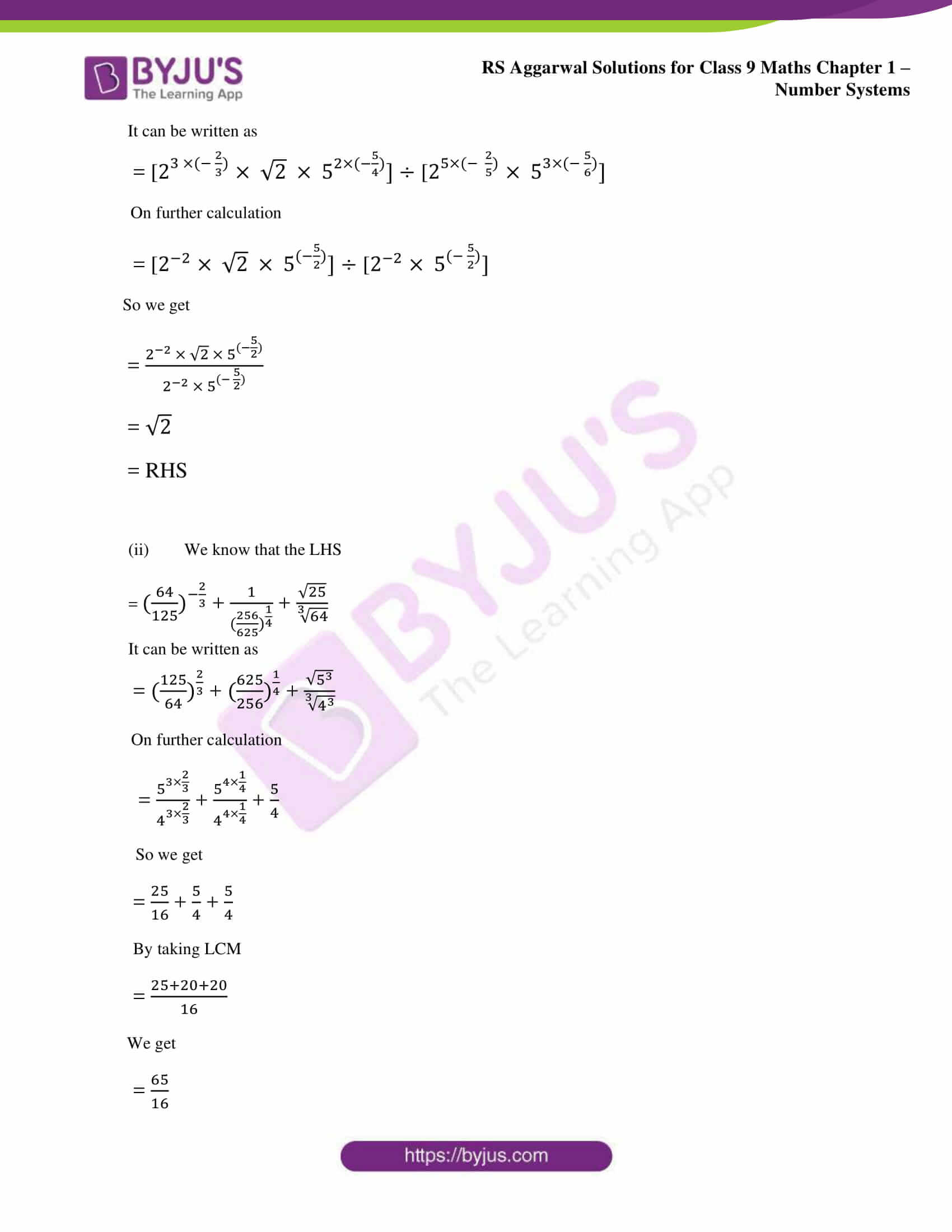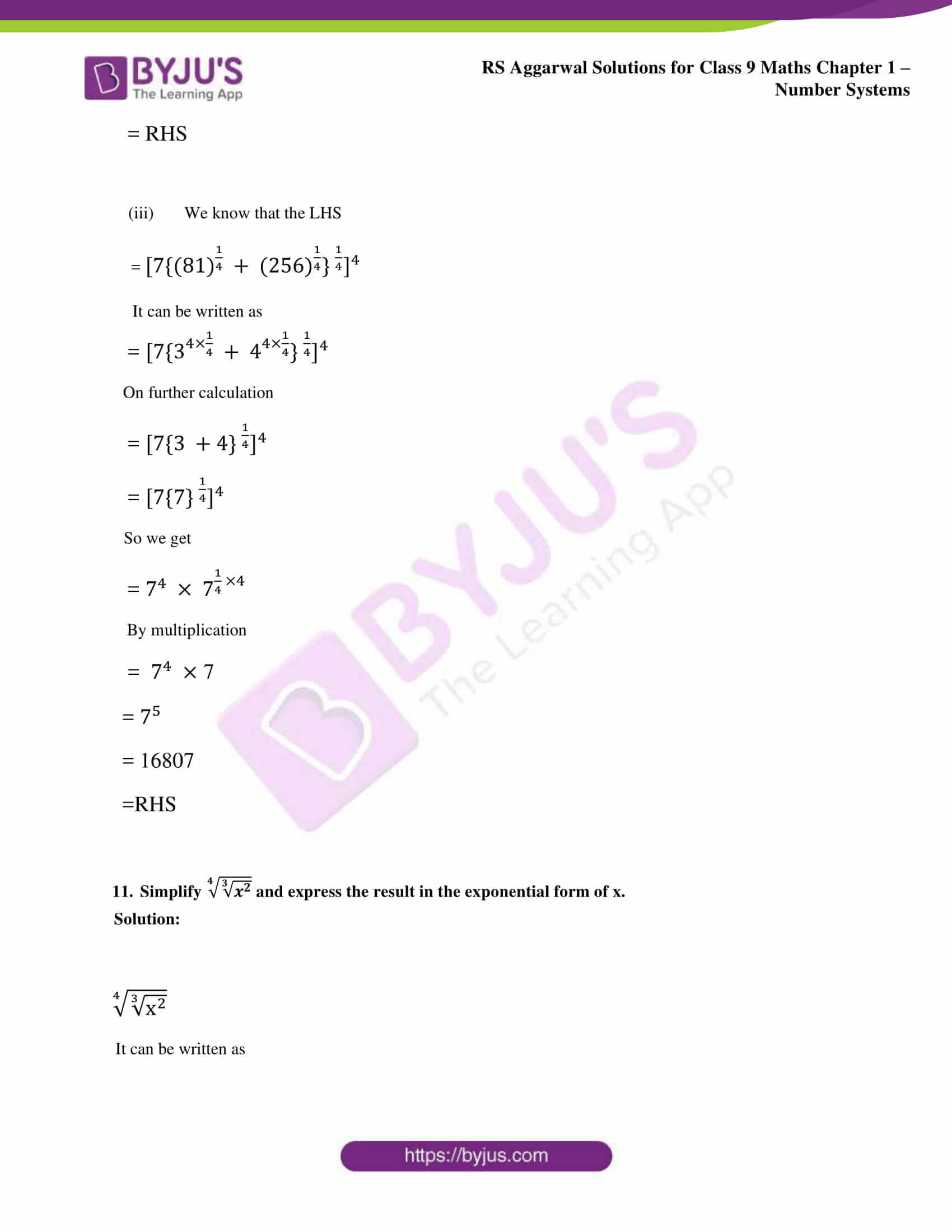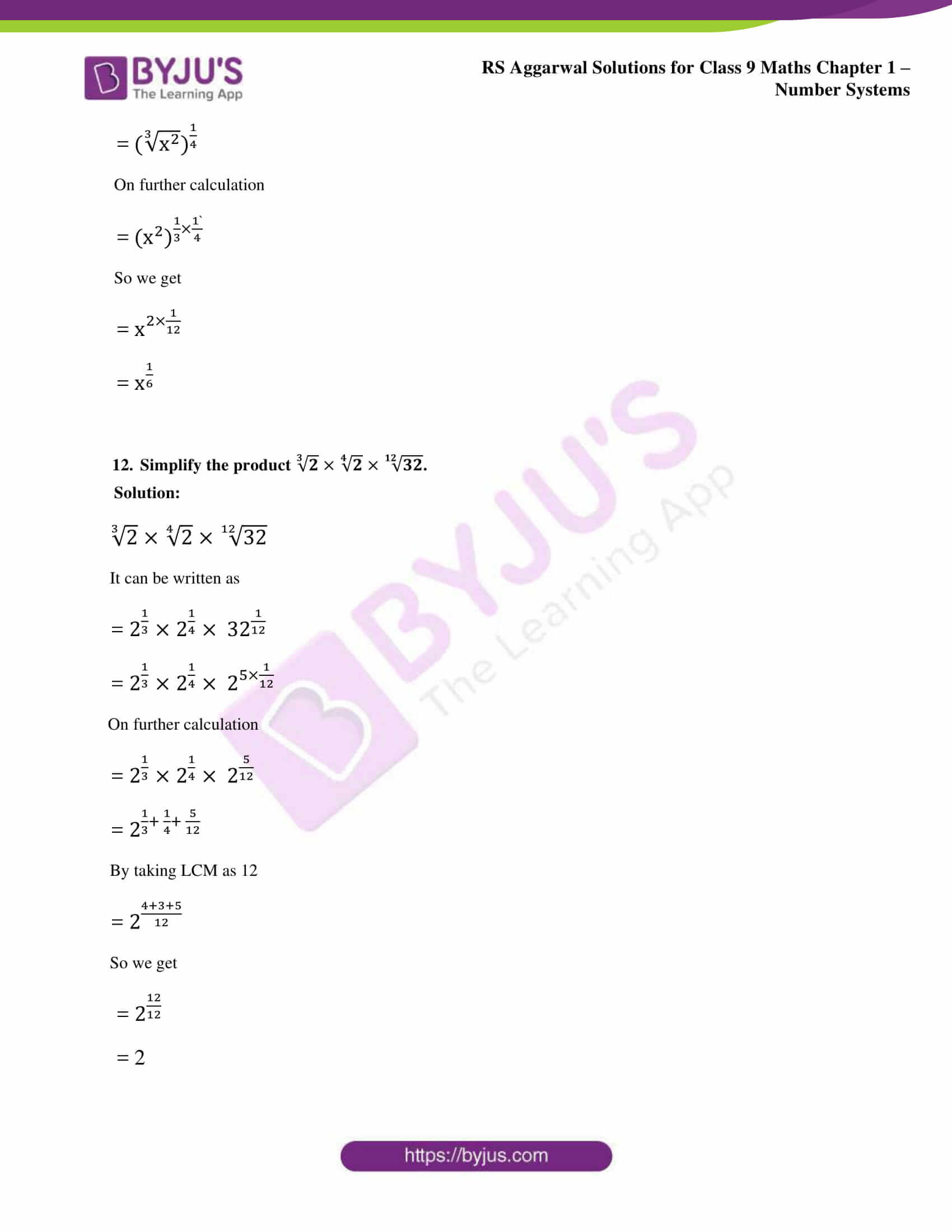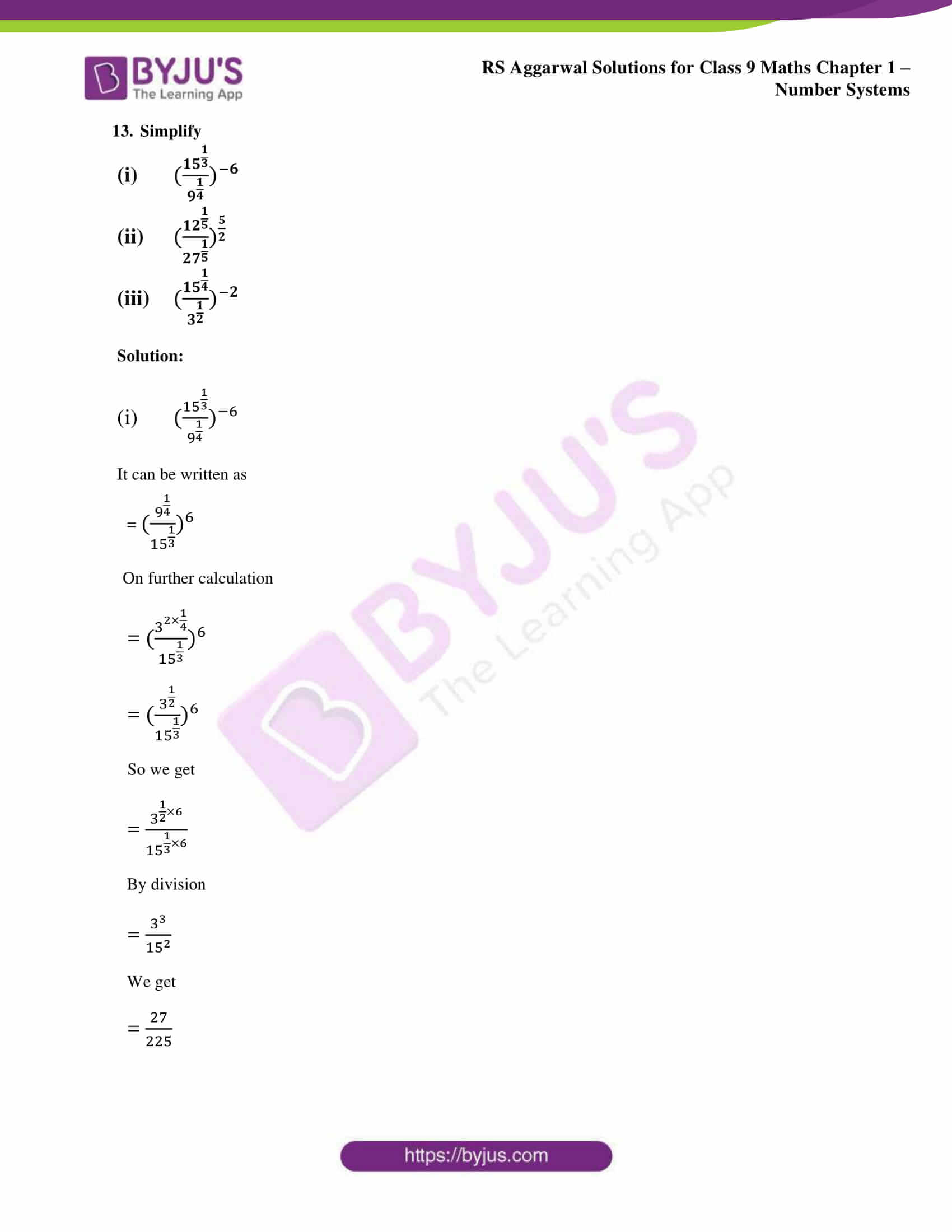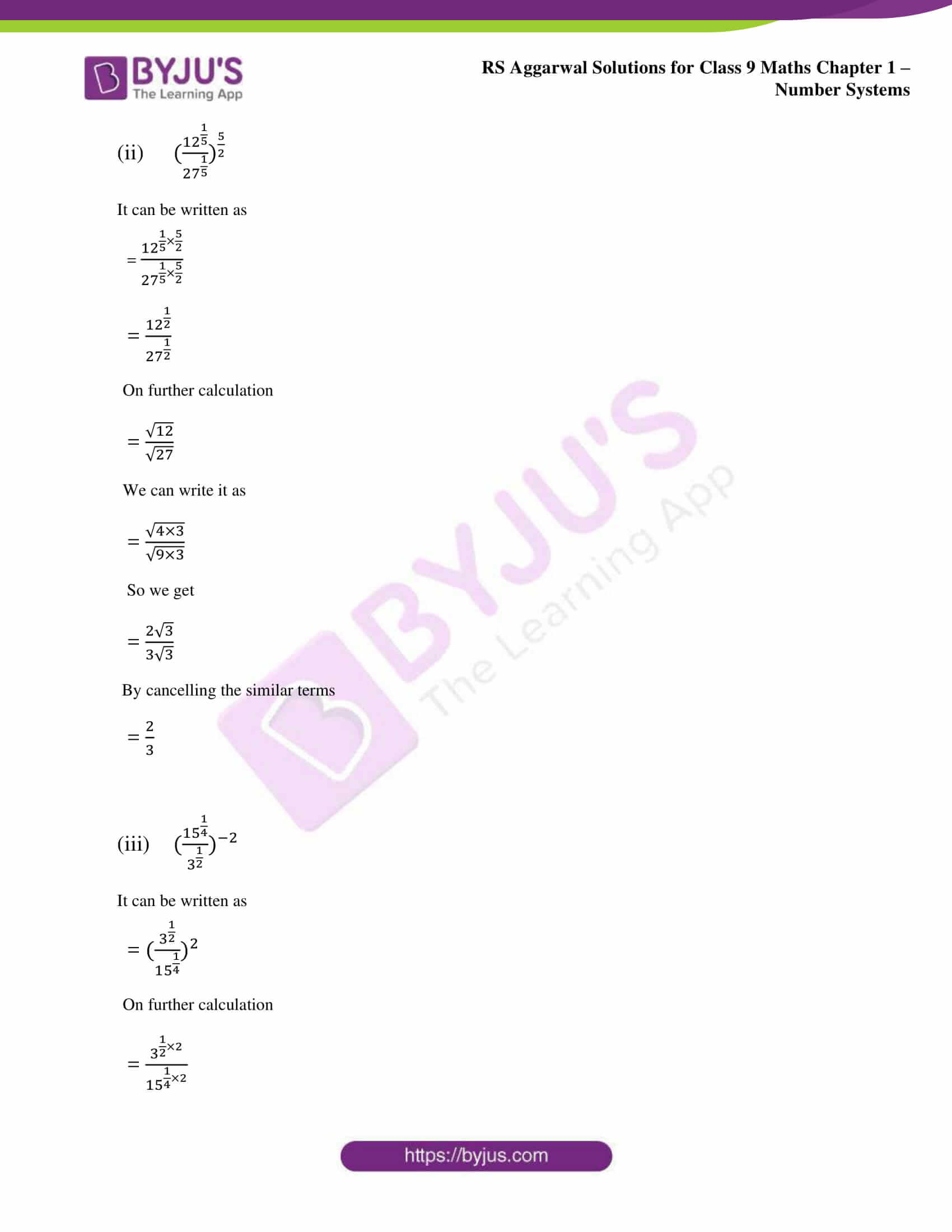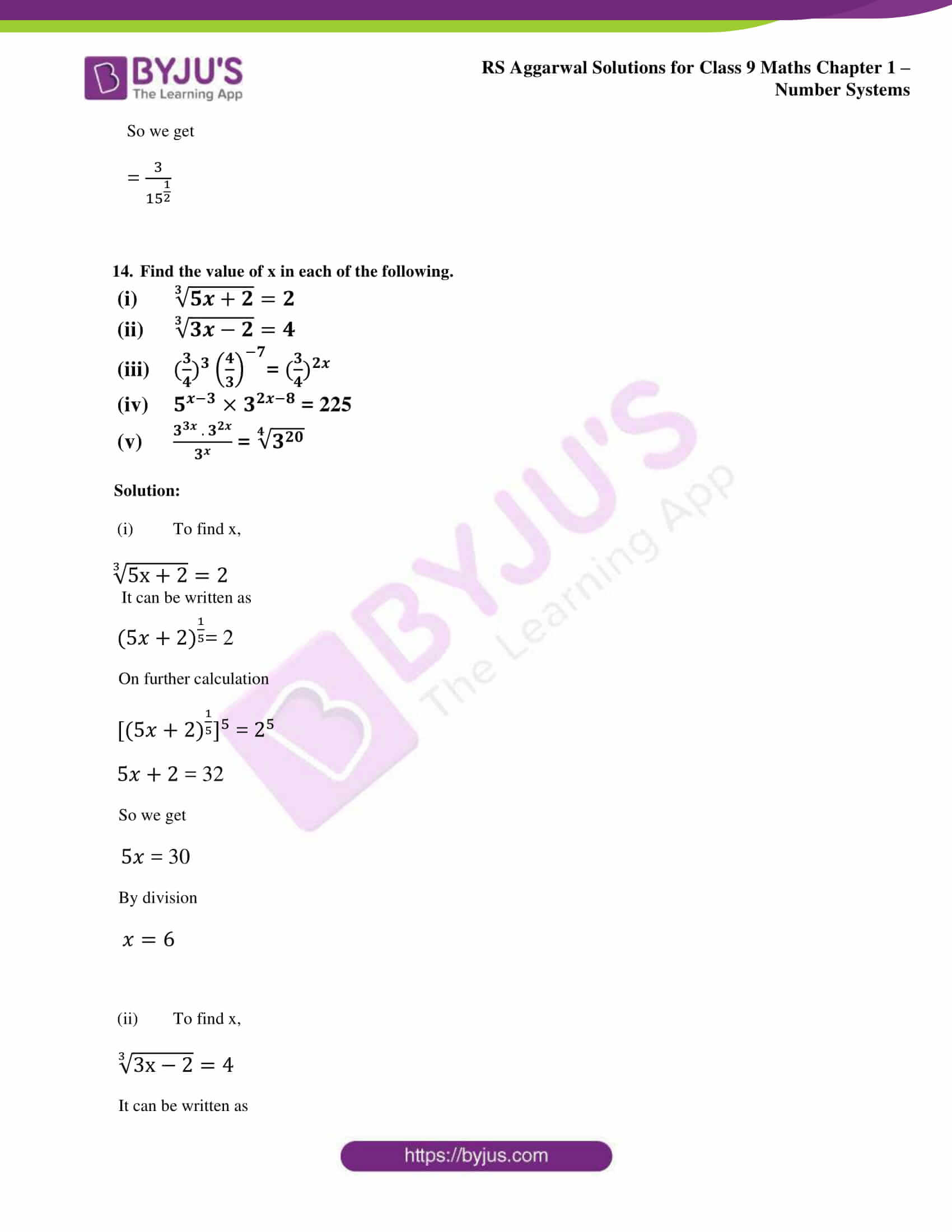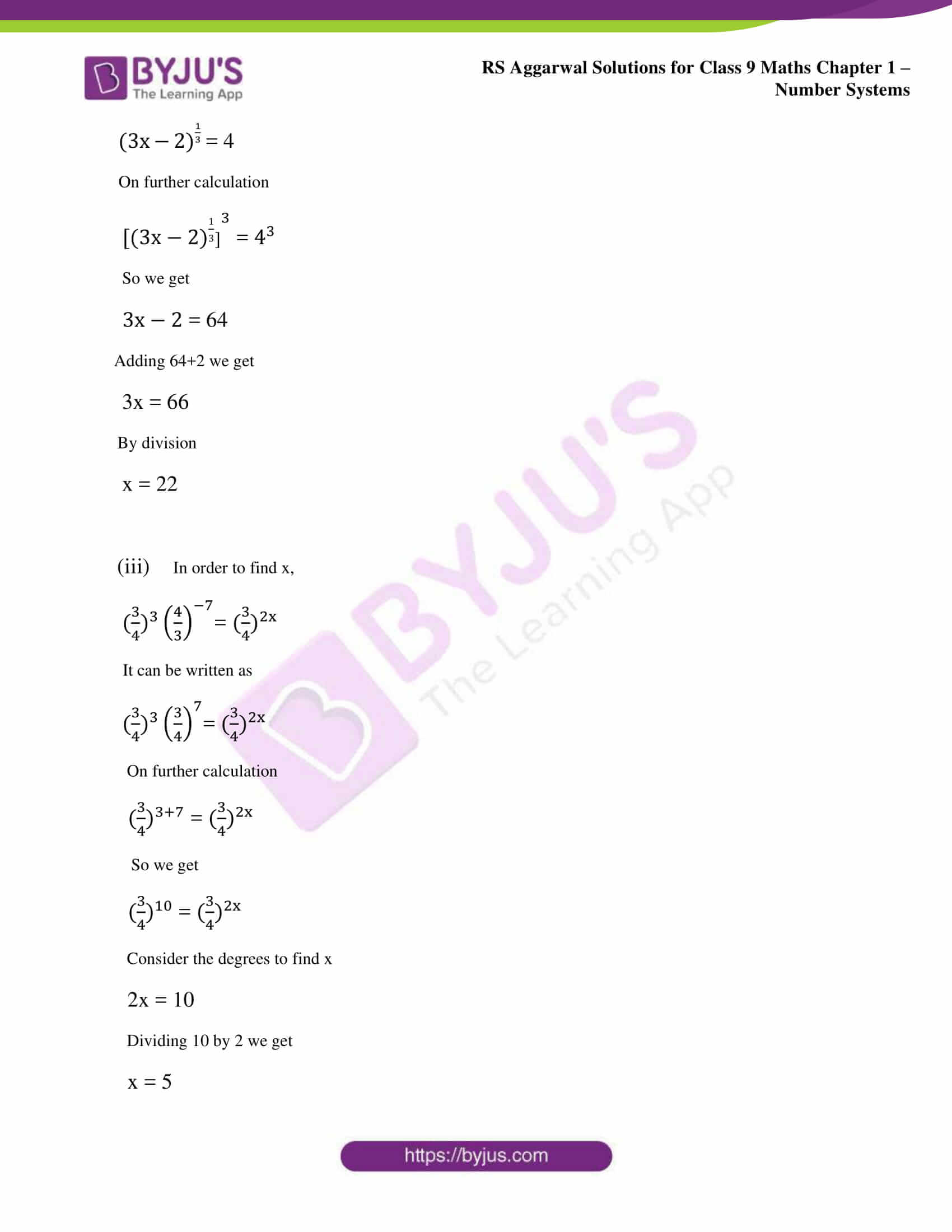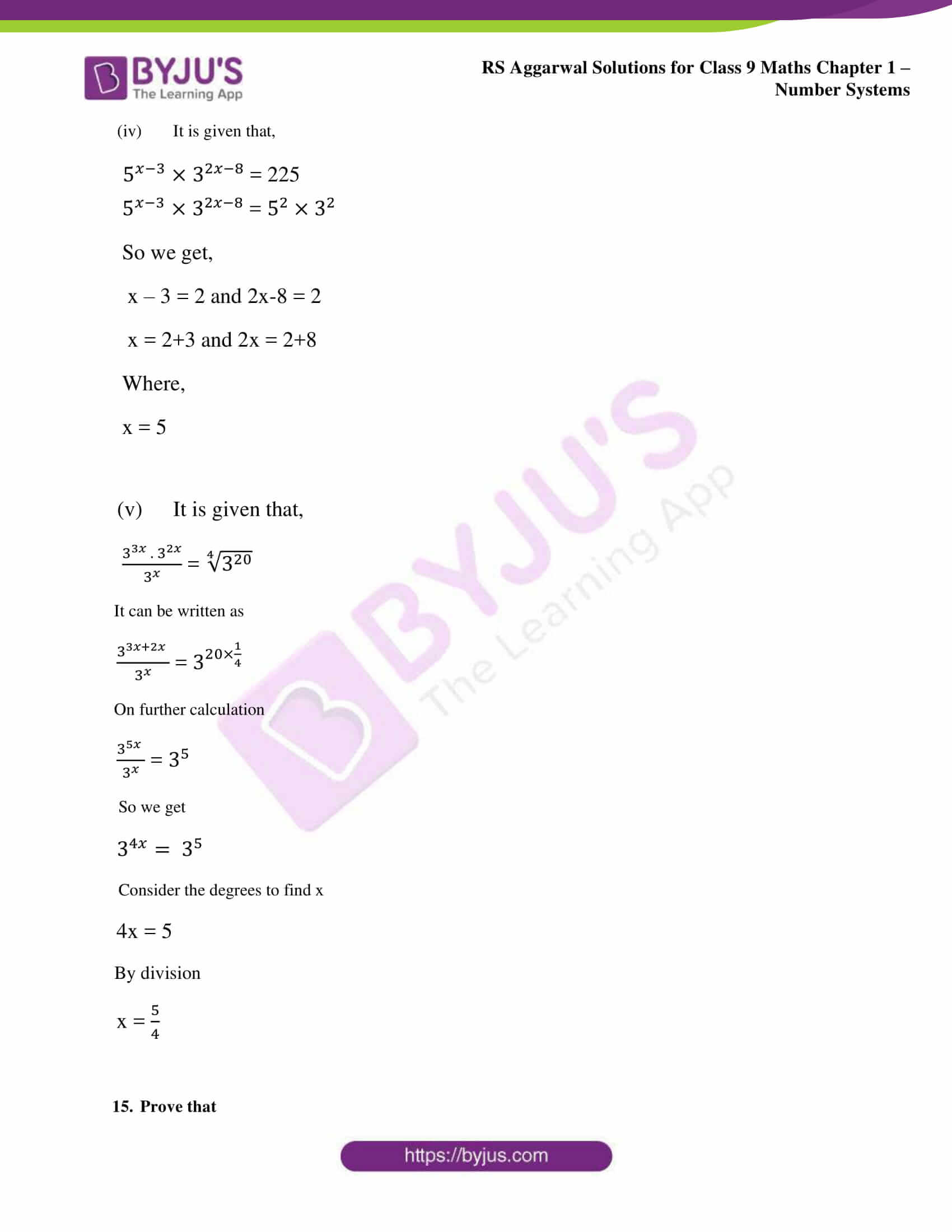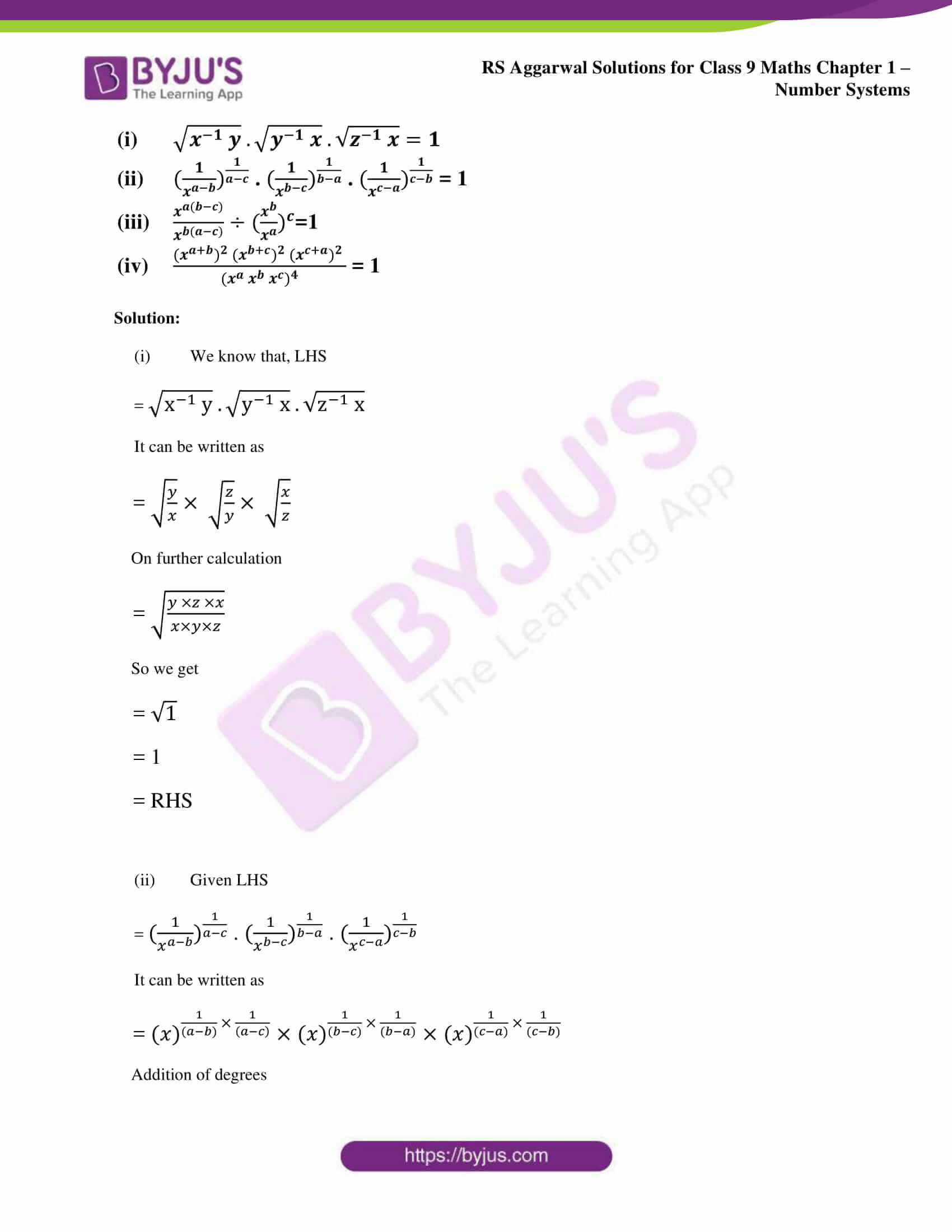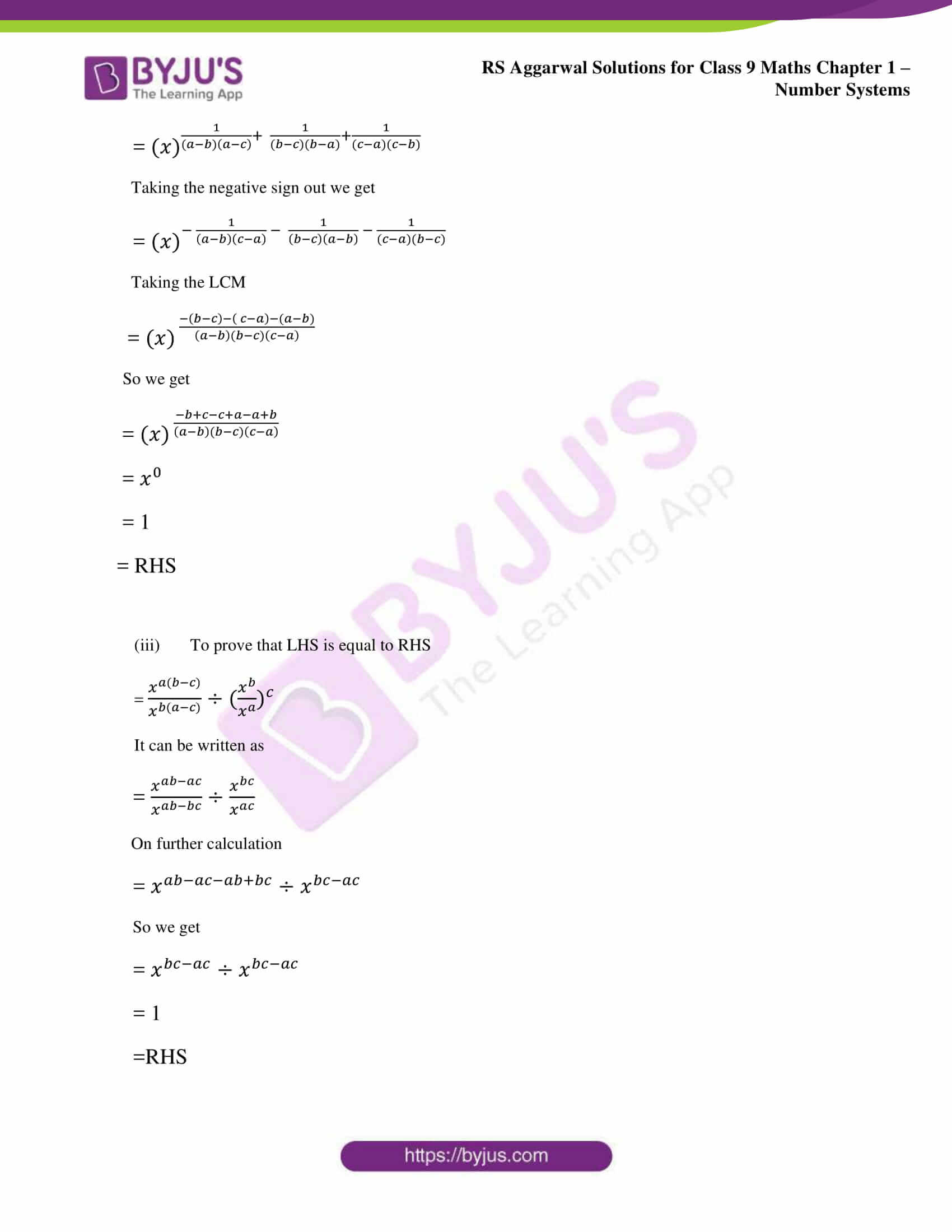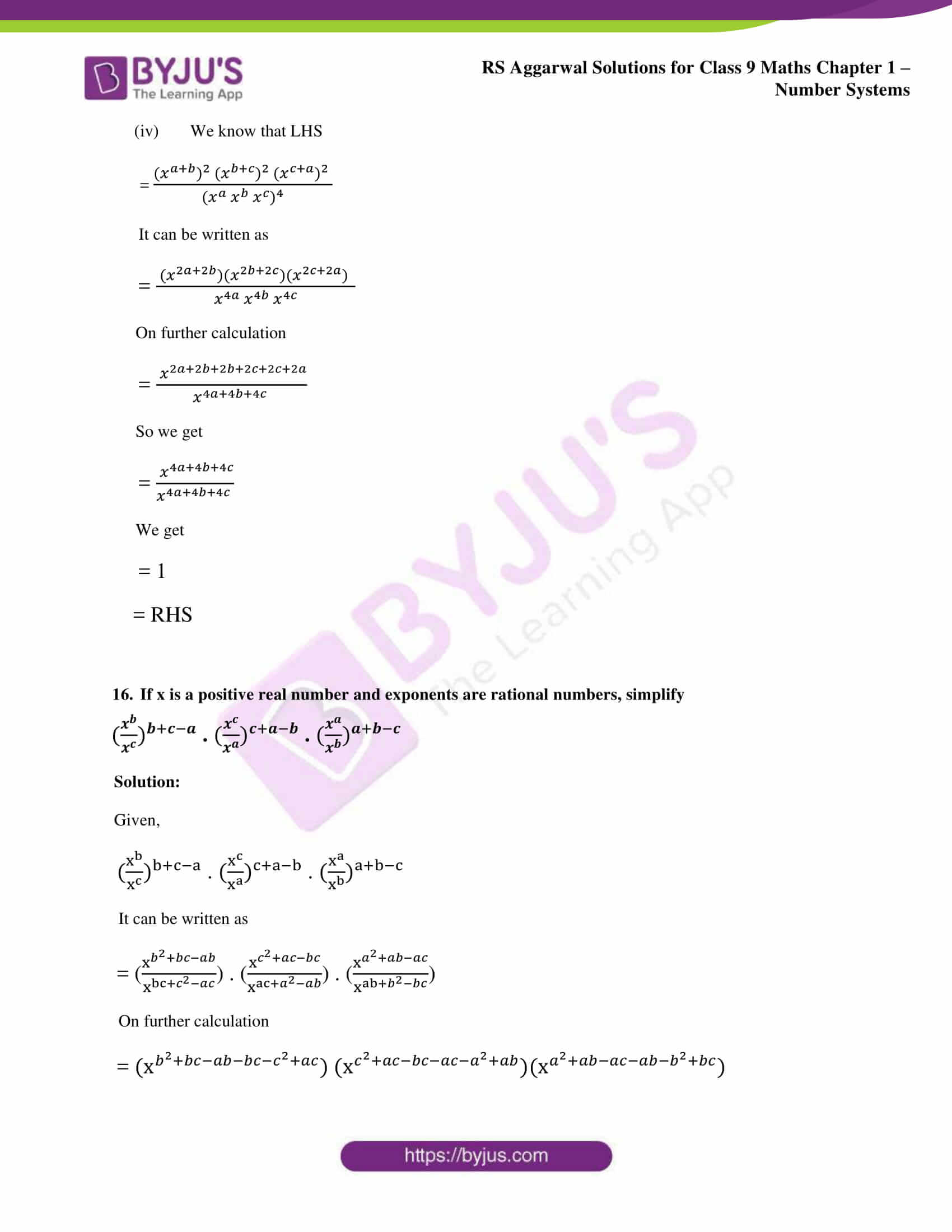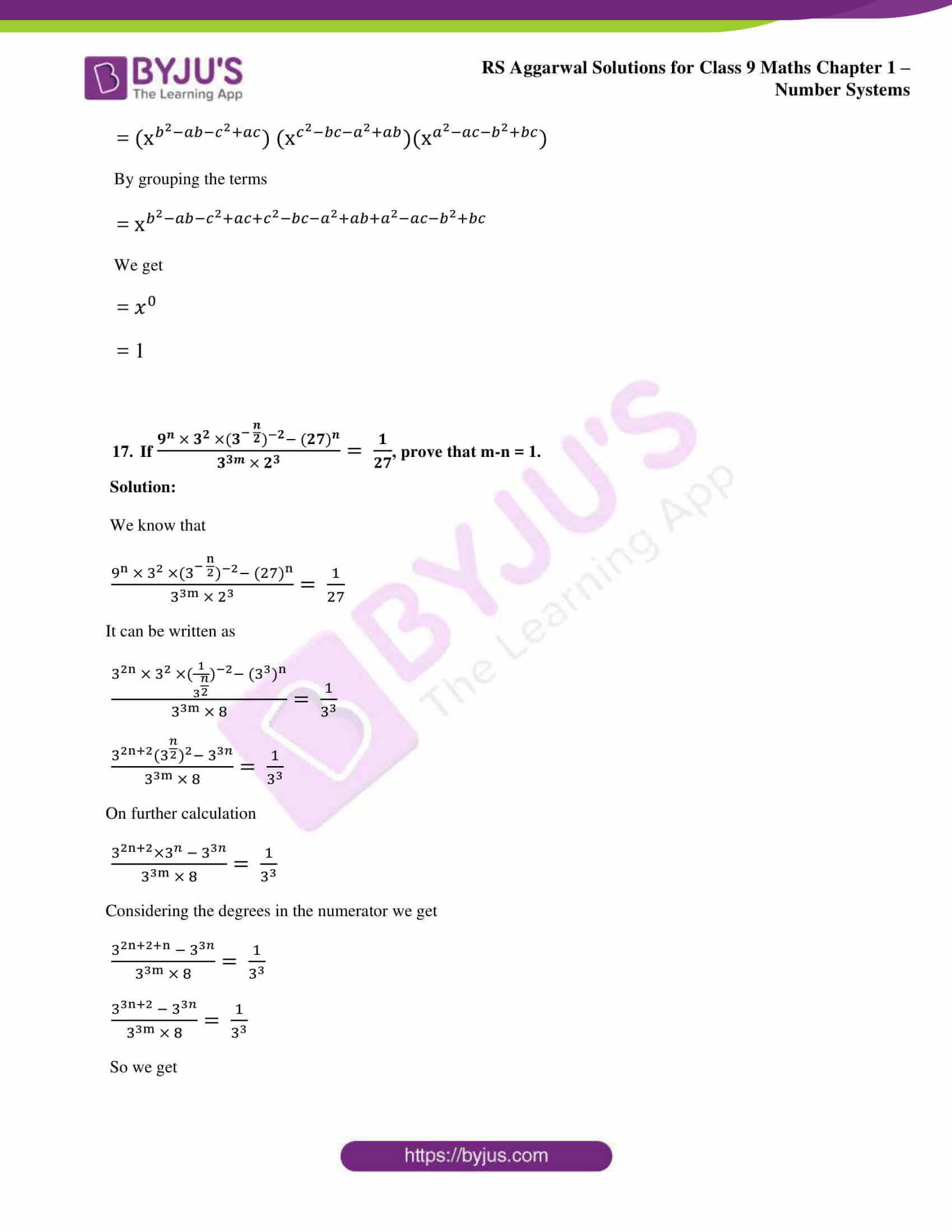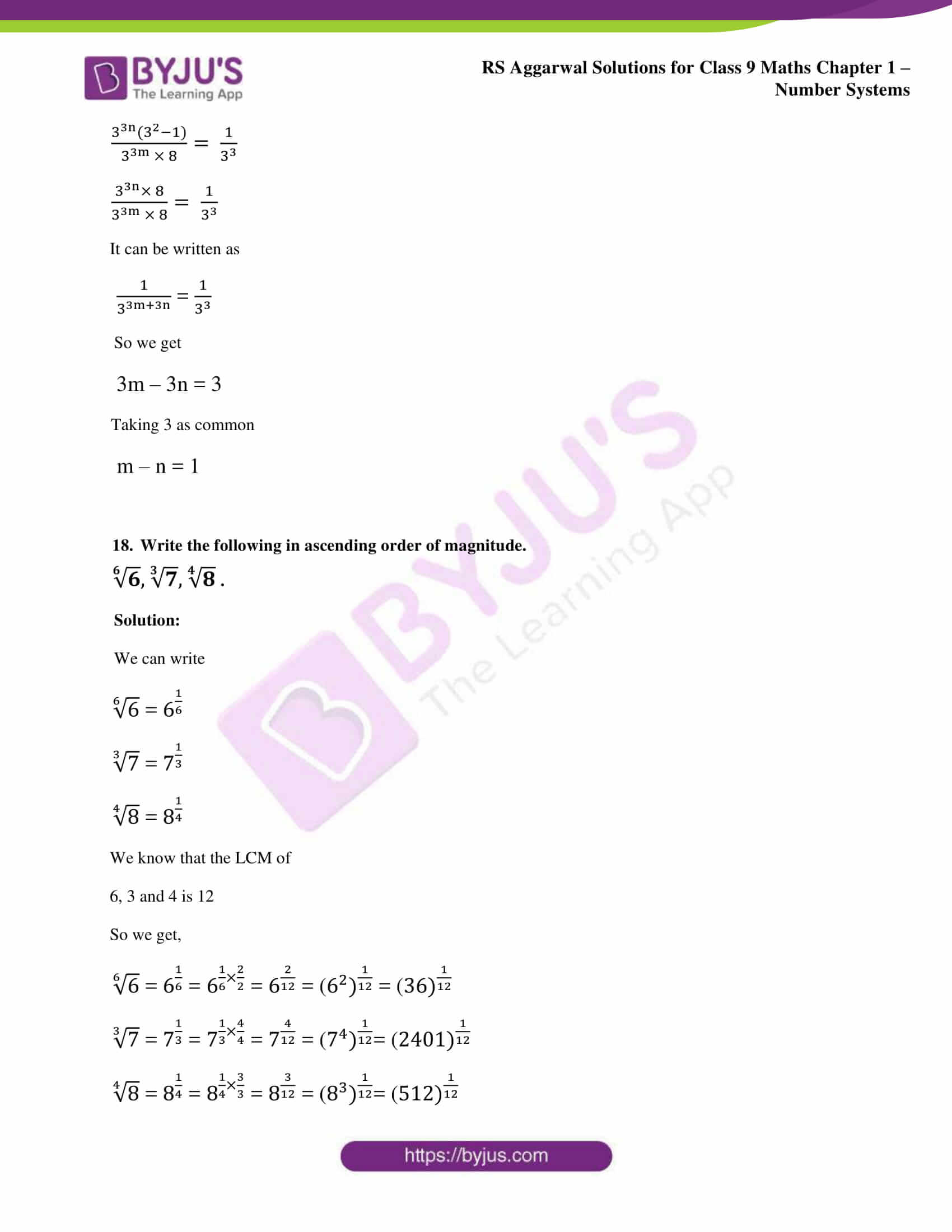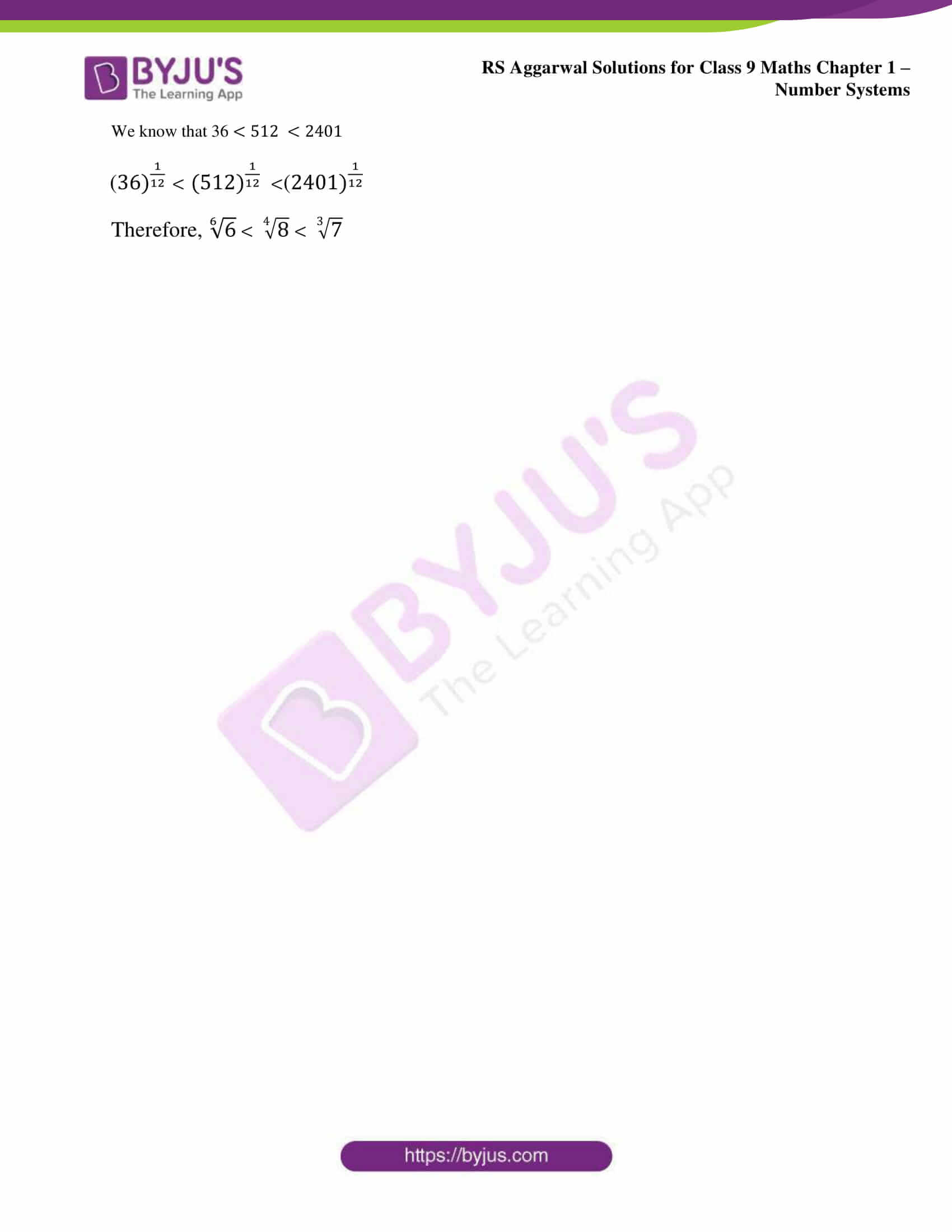### Access other exercise solutions of class 9 Maths Chapter 1: Number Systems

Exercise 1A Solutions 9 Questions

Exercise 1B Solutions 5 Questions

Exercise 1C Solutions 14 Questions

Exercise 1D Solutions 8 Questions

Exercise 1F Solutions 25 Questions

### RS Aggarwal Solutions Class 9 Maths Chapter 1 – Number Systems Exercise 1G

RS Aggarwal Solutions Class 9 Maths Chapter 1 Number Systems Exercise 1G has a set of problems with solutions which is based on the Laws of Exponents and some of the important results.

### Key features of RS Aggarwal Solutions for Class 9 Maths Chapter 1: Number Systems Exercise 1F

• Many number of examples before solving the main problems will help students have a better idea of concepts.
• The problems solved in the RS Aggarwal textbook are based on the CBSE syllabus for Class 9.
• Listing of formulas and concepts of importance before each exercise helps students perform better in the exam.
• RS Aggarwal Solutions in PDF format can be used by the students in order to obtain meritorious position in the exam.